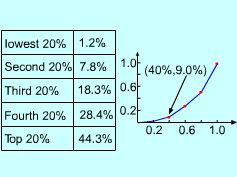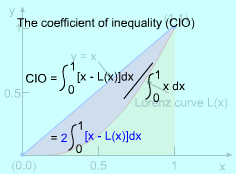Ch 8. Integration Techniques Multimedia Engineering Math Integration byParts Trig. IntegralsTrig. Subst. Fractions& Substitutions ApproximateIntegration
 Chapter 1. Limits 2. Derivatives I 3. Derivatives II 4. Mean Value 5. Curve Sketching 6. Integrals 7. Inverse Functions 8. Integration Tech. 9. Integrate App. 10. Parametric Eqs. 11. Polar Coord. 12. Series Appendix Basic Math Units Search eBooks Dynamics Fluids Math Mechanics Statics Thermodynamics Author(s): Hengzhong Wen Chean Chin Ngo Meirong Huang Kurt Gramoll ©Kurt GramollMATHEMATICS - CASE STUDY IntroductionThe Lorenz CurveThe Coefficient of Inequality Norman is an economist who works on the distribution of income between households in a country. The cumulative distribution he used is called a Lorenz curve. A Lorenz curve goes from 0 to 1 and includes the points (0, 0) and (1, 1). The points on this Lorenz curve are determined by ranking all households by income and then computing the percentage of households whose income is less than or equal to a given percentage of the total income of the country. If a given percent of the households receive the same percent of income, the Lorenz curve is the line y = x and is called absolute equality. The coefficient of inequality is used to describe the difference between the Lorenz curve and the absolute equality curve. What is know: The income distribution for a given country is represented by a Lorenz curve defined by the equation L(x) = 5x3/(x2 + x +3) The coefficient of inequality is given aswhere L(x ) is the Lorenz curve. Questions Find the coefficient of inequality (COI) of the give Lorenz curve. Approach L(x) is an improper rational function. Use the preliminary step of dividing to simplify L(x) to a proper rational function. x2 + x +3 is an irreducible quadratic factor. Use the method introduced in case 3 of theory to integrate it.